6351.0.55.001 - Wage Price Index: Concepts, Sources and Methods, 2012
Latest ISSUE Released at 11:30 AM (CANBERRA TIME) 28/11/2012
Page tools:Print AllRSSSearch this Product
Contents >> Chapter 4 - Price Index Theory

CHAPTER 4 – PRICE INDEX THEORY

INTRODUCTION

4.1 This chapter describes the price index theory underpinning the WPI. A more comprehensive exploration of price index theory can be found in corresponding chapters of Consumer Price Index: Concepts, Sources and Methods, 2011 (cat. no. 6461.0).

 4.2 As the name indicates, the WPI is a price index. Price indexes provide a convenient and consistent way of presenting price movement information for items. In the WPI, the items of interest are jobs. However, the general term "item" is used throughout the chapter as it is consistent with general price index theory. 4.3 An index number on its own has little meaning. The value of a price index stems from the fact that index numbers for any two periods can be used to directly calculate price change between those periods. For example, the WPI All sectors Australia index number of 110.5 in the December quarter 2011 compared to the index number of 106.6 in the December quarter 2010 informs the user that Australian wage prices have risen by 3.7% in the 12 month period described (ie [110.5 - 106.6]/106.6 x 100).

THE CONCEPT OF A PRICE INDEX

Comparing prices

4.4 There are many situations where there is a need to compare two (or more) sets of observations on prices. For example, a business may want to compare the price of labour today with that from an earlier period. An employer might want to compare the increase in wages in their organisation with those across the industry. An economist may be interested in understanding wage pressures across the economy.

4.5 In some situations the price comparisons might only involve a single item. Here it is simply a matter of directly comparing the two price observations. In other circumstances the required comparison is of prices across a range of items. In this instance a method is required for combining the prices allowing for the fact that they have many different tasks or quantities of measurement. This is where price indexes play an extremely useful role.

The basic concept

4.6 Price indexes such as the WPI enable prices for a common item or group of items to be compared at different points in time. In order to compare the sets of prices it is necessary to designate one set the ‘reference’ set and the other the ‘comparison’ set. By convention, the reference price set is used as the base (or first) period for constructing the index and is given an index value of 100.0. For example, suppose for a single item the average of prices in set 1 was \$15 and for set 2 it was \$30. Designating set 1 as the reference set gives an index of 200.0 (30/15×100) for the comparison set while designating set 2 as the reference set gives an index of 50.0 (15/30×100) for the comparison set.

4.7 The most common comparison is between sets of prices at two points in time (temporal indexes). The points in time can be adjacent (this quarter and the previous quarter) or many periods apart (this year and ten years earlier). Typically the method is to nominate one set of prices as the reference period and to revalue the quantities of items purchased in the base period by prices in the second (or comparison) period. The ratio of the revalued comparison period to the value of the reference period provides a measure of the price change between the two periods.

4.8 The handling of quantity changes that occur in response to changes in relative prices is fundamental to price index construction. Changes in the relative importance of items being priced can have a significant effect on index movements.

MAJOR INDEX FORMULAS

4.9 In presenting index number formulas a simple starting point is to compare two sets of prices. Consider price movements between two time periods, where the first period is denoted as period 0 and the second period as period t (period 0 occurs before period t). In order to calculate the price index, the quantities need to be held fixed at some point in time. The initial question is what period should be used to determine the quantities. The options are to use:

(i)The quantities of the first (i.e. earlier) period. This approach answers the question ‘how much would it cost in the second period, relative to the first period, to purchase the same quantities of items as purchased in the first period’. Estimating the cost in the second period’s prices simply requires multiplying the quantities of items purchased in the first period by the prices that prevailed in the second period. A price index is obtained from the ratio of the revalued cost using the second period prices to the cost in the first period. This approach was proposed by Laspeyres in 1871 and is referred to as a Laspeyres price index. It may be represented, with a base of 100.0, as:

(4.1)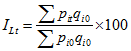where:
p = price
q = quantity
i = item.

(ii) The quantities of the second (or more recent) period. This approach answers the question ‘how much would it have cost in the first period, relative to the second period, to purchase the same quantities of items as purchased in the second period'. Estimating the cost in the first period simply requires multiplying the quantities of items purchased in the second period by the prices prevailing in the first period. A price index is obtained from the ratio of the cost in the second period to the revalued cost using the first period’s prices. This approach was proposed by Paasche in 1874 and is referred to as a Paasche price index. It may be represented, with a base of 100.0, as:

(4.2)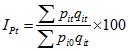(iii) A combination (or average) of quantities in both periods. This approach tries to overcome some of the inherent difficulties of using quantities of items fixed at either point in time. In the absence of any firm indication that either period is the better to use as the base or reference, then a combination of the two is a sensible compromise. In practice this approach is most frequent in:

a) the Fisher Ideal price index, which is the geometric mean of the Laspeyres and Paasche indexes:

(4.3)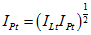b) the Törnqvist price index, which is a weighted geometric mean of the price relatives where the weights are the average shares of total values in the two periods, that is:

(4.4)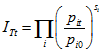where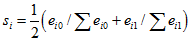is the average of the expenditure shares for the ith item in the two periods.

4.10 The Fisher Ideal and Törnqvist indexes are often described as ‘symmetrically weighted indexes’ because they treat the weights from the two periods equally.

4.11 All price indexes in the ABS use the Laspeyres price index formula. This is largely because the weights used are held fixed at some earlier base period. On the other hand the Paasche, Fisher Ideal and Törnqvist formulas all require current period weights. Timely information of this nature is often very difficult to obtain.

4.12 It should be noted that the different index formulas produce different index numbers and thus different estimates of price movement. Typically the Laspeyres formula will produce a higher index number than the Paasche formula, with the Fisher Ideal and the Törnqvist index numbers falling between the two. In other words the Laspeyres index will generally produce a higher measure of price increase (or lower decrease) than the other formulas and the Paasche index a lower measure of price increase (or larger decrease).

4.13 Only the Laspeyres price index formula will be considered from this point. Further information regarding price index theory can be found in Consumer Price Index: Concepts, Sources and Methods, 2011 (cat. no. 6461.0).

4.14 The Laspeyres formula above is expressed in terms of quantities and prices. In practice, quantities might not be observable or meaningful. Thus in practice the Laspeyres formula is typically estimated using expenditure shares to weight price relatives - this is numerically equivalent to the formula (4.1) above.

4.15 To derive the price relatives form of the Laspeyres index, multiply the numerator of equation (4.1) by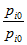and rearrange to obtain:

(4.5)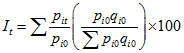where the term in parentheses represents the expenditure share of item i in the reference (or, more commonly labelled, base) period. Let:

(4.6)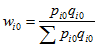then the Laspeyres formula may be expressed as:

(4.7)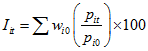where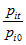is the price relative for the ith item.

4.16 The important point to note here is that if price relatives are used then value (or expenditure) weights must also be used. On the other hand, if prices are used directly rather than in their relative form, then the weights used must be quantities.

 Table 4.1: COMPILING PRICE INDEXES OVER TWO PERIODSPrice0 Quantity0 Expenditure0 Expenditure shares0 Price relativesItem p i0 q i0 e i0 w i0 p i0/p i01 2.50 2 000 5 000 0.4310 1.00002 3.00 500 1 500 0.1293 1.00003 4.50 200 900 0.0776 1.00004 1 500.00 2 3 000 0.2586 1.00005 30.00 40 1 200 0.1035 1.0000Total11 600 1.0000Pricet Quantityt Expendituret Expenditure sharest Price relatives Item p it q it e it w it p it/p i0 1 2.75 2 000 5 500 0.4532 1.1000 2 4.00 450 1 800 0.1483 1.3333 3 6.50 130 845 0.0696 1.4444 4 1 000.00 3 3 000 0.2472 0.6667 5 33.00 30 990 0.0817 1.1000 Total12 135 1.0000INDEX NUMBERS Index formula Period 0 Period t Laspeyres 100.0 104.5

The following illustrates the Laspeyres index number calculation:

Laspeyres = 100.0 x [(0.4310 x 1.1000) + (0.1293 x 1.3333) + (0.0776 x 1.4444) + (0.2586 x 0.6667) + (0.1035 x 1.1000)].

GENERATING INDEX SERIES OVER MORE THAN TWO TIME PERIODS

4.17 Most users of price indexes require a continuous series of index numbers at specific time intervals. There are two options for applying the Laspeyres formula when compiling a price index series:

(i) select one period as the base and separately calculate the movement between that period and the required period, which is called a ‘fixed base’ or ‘direct’ index;
(ii) calculate the period to period movements and 'chain' these (i.e. calculate the movement from the first period to the second and the second to the third, with the movement from the first period to the third obtained as the product of these two movements).

4.18 The calculation of direct and chain indexes over three periods (0, 1, and 2) using observations on items as used in Table 4.1 is shown in Table 4.2. The procedures can be extended to cover many periods.

 Table 4.2: CONSTRUCTING PRICE INDEX SERIESItem Period 0 Period 1 Period 2PRICE (\$)1 2.50 2.75 3.502 3.00 4.00 4.503 4.50 6.50 7.504 1 500.00 1 000.00 1 000.005 30.00 33.00 40.00EXPENDITURE SHARE1 0.4310 0.45322 0.1293 0.14833 0.0776 0.06964 0.2586 0.24725 0.1035 0.0817INDEX NUMBERSIndex methodologyPeriod 0 to 1 100.0 104.5Period 1 to 2100.0 117.0Direct 100.0 104.5 123.7Chain 100.0 104.5 122.3In this example, the Laspeyres Chain Index for period 2 is calculated as follows:

Indext=2 = (104.5/100) x (117.0/100) x 100
= 122.3.

THE APPROACH USED IN THE WAGE PRICE INDEX

4.19 In the discussion above it was shown how various price index formulae reflect different options as to the period in which quantities are determined.

4.20 The WPI utilises the Laspeyres index methodology since it is not practical to calculate current weights every period, as is required by other methods.

4.21 The weights for the WPI are updated regularly to reflect changes in the composition of jobs and indexes produced with the new weights are chain linked to those produced with the earlier weights.

4.22 This chapter has outlined the general price theory. The remainder of this Concepts, Sources and Methods publication is dedicated to showing how these concepts are practically applied to the WPI.